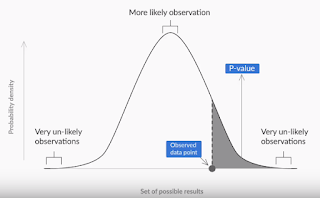p value from z score - p value formula

# P value from Z score

### P value method - P value formula

In this post we would look at how to find P-value from Z score. This is the P value method of Hypothesis testing.

The steps of P-value method is just the opposite of Critical Value method that we had discussed in this earlier post.

In this method, we first keep the significance level aside.
It is used only in the end to compare and make a decision.
Here we start with the sample mean (recall how in Critical Value method we kept sample mean aside).

Now, using this sample mean we calculate the value of Zc from the following formula:

Zc = (X - U) / ( Sigma /Sqrt(n) )

X is the given sample mean,
U is the mean claimed in the Null Hypothesis
Sigma is the standard deviation of the sample
N is the sample size.

Once you know the Zc, all we need to do is use the Z-table to find the corresponding cumulative probability.
(ie. The percentage of area covered in the graph till that point of Zc).
This percentage is the P-value that we were trying to find.

Keep in mind, if it is a two tailed test, then this percentage has to be multiplied with 2 to get the P-value.

Now, to make a decision all we need to do is compare this P-value with the Significance level.

If P-value is less than the significance level, then you reject the Null hypothesis.
Otherwise, we fail to reject the null hypothesis.

What is the conceptual understanding of this P-value?

The higher the p-value, the higher is the probability of failing to reject a null hypothesis.
And the lower the p-value, the higher is the probability of the null hypothesis being rejected.
As you can observe from the image below, the higher the P-value the more close to the center of the graph we would be and hence more close to our claim in Null hypothesis and thus less chance of rejecting it.
On the other hand, lower the P-value the higher the chances of rejecting the null hypothesis since we would be more close to the ends of the graph.